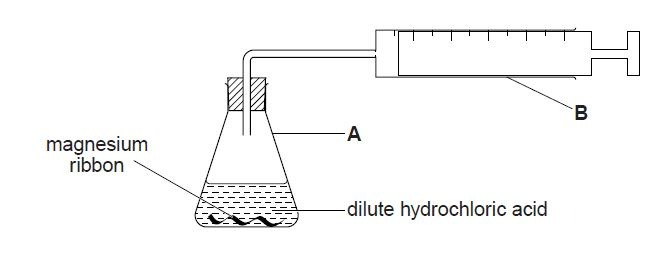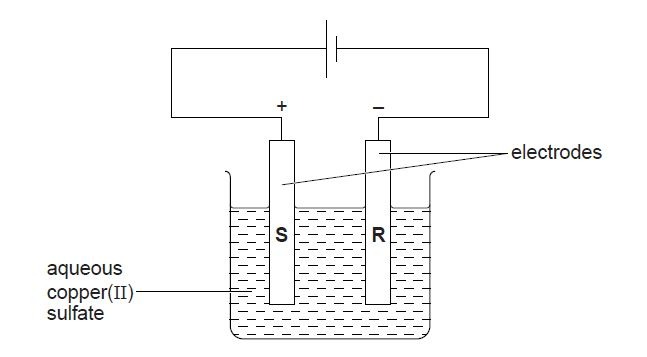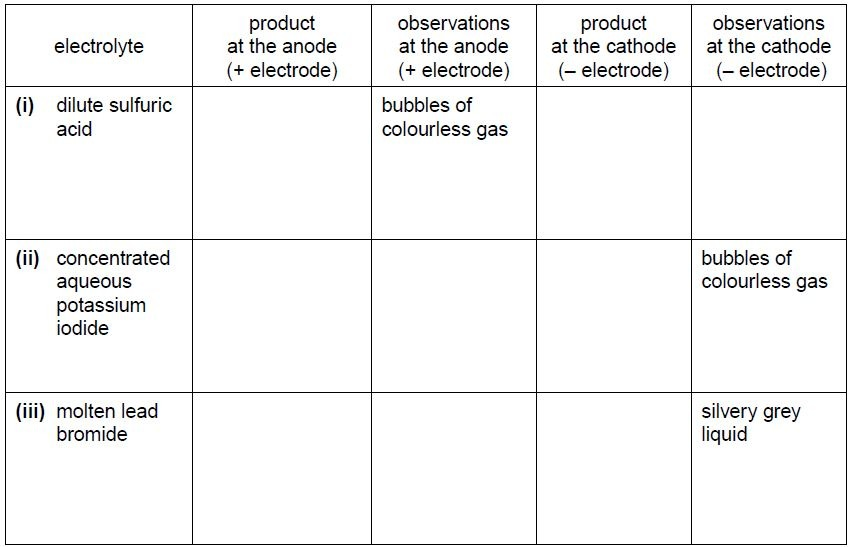# WAEC GCE Chemistry Practical Questions and Answers 2022/2023

The Waec Gce chemistry practical 2022 alternative question and answers for exam have been released. The General Certificate Examination (GCE) for WAEC Chemistry alternative to practical paper will be written on Monday, 7th February 2022 from 2:00 pm to 3:30 pm.

We made this post to highlight samples of the Specimens, alternative to practical graphs and calculations that was used in the Chem Practical Examination past questions and syllabus.

Always keep in mind that each and every operation receives a grade. As a result, when reporting and presenting your practical outcomes, make certain that you do not miss any steps.

The Chemistry Practical question paper will be divided into two portions, each of which will include general Chemistry questions, as follows:

1. Quantitative Analysis
2. Qualitative Analysis

Titration and ion testing are two of the procedures. You will be required to conduct an experiment and submit your findings as well as your inferences and observations. In terms of content, you will not be far off the WAEC Chemistry Specimen that was sent to your school.

### Alternative WAEC GCE Chemistry Practical Questions 2022

Paper 3 (Alternative To Practical)

1. A student adds a known mass of magnesium ribbon to 100 cm3 of dilute hydrochloric acid (an excess) in the apparatus shown below. Hydrogen gas is evolved. The time taken to collect 48 cm3 of gas at room temperature and pressure is measured.(a) (i) Name apparatus A.
(ii) Name apparatus B.
(b) (i) Calculate the number of moles of hydrogen in the 48 cm3 of gas.
[1 mole of any gas occupies 24 000 cm3 at room temperature and pressure.]

(ii) Use the equation below to deduce the mass of magnesium used in the experiment to produce 48 cm3 of hydrogen at room temperature and pressure. [Ar : Mg, 24]
Mg + 2HCl MgCl2 + H2
(c) Give a test for hydrogen gas.

(d) What is the effect on the time taken to collect the same volume of gas if,
(i) large lumps of the same mass of magnesium are used instead of magnesium ribbon,
(ii) the reaction is carried out at a higher temperature?

2. (a) A student investigates the electrolysis of aqueous copper(II) sulfate using graphite electrodes and the apparatus shown below.(i) What is observed at electrode R?
(ii) Construct the equation for the reaction taking place at electrode R.
(iii) When graphite electrodes are used a colour change is seen in the solution. What is this colour change? Explain why it happens.
(iv) When graphite electrodes are used, a gas is evolved at one of the electrodes. Name the gas and give a test for the gas.
(v) If the graphite electrodes are replaced with copper electrodes, no colour change is seen on the electrolysis of the solution. Explain why.

(b) The student does more experiments using the apparatus in (a) with graphite electrodes
but, in each case, using a different electrolyte.
Complete the table below.The details of the WAEC Chem practical drawings and answers will be made available to you candidates as soon as it is released. Keep following this page and make sure you bookmark this site for reference purposes.

If you have any questions, endeavour to use the comment box below…

### Practice Questions: WASSCE GCE Chemistry Possible Questions 2022

The questions below are strictly for practice, not the 2022 Chemistry expo.

All your burette readings (initials and final), as well as the size of your pipette, must be recorded but no account of experimented procedure is required. All calculations must be done in your booklet.

1. A is O.200 moldm3 of HCI. C is a solution containing 14/3g of Na2CO3. x H2O in 500 cm3 of solution. (a) Put A into the burette and titrate it against 20.0 cm3 or 25.0 cm3 portions of C using methyl orange as indicator. Repeat the titration to obtain Consistent titre values. Tabulate your results and calculate the average volume of A used. The equation for the reaction is: Na2CO3 x H2O + 2HCL(aq) → 2NaCI(aq) + CO2(g) + (x+1) H2O(I).

(b) From your results and the information provided. Calculate the: (i) concentration of C in moldm-3; (ii) concentration of C in gdm-3; (iii) molar mass of Na2CO3, xH2O; (iv) the value of x in Na2CO3 xH2O. [H = 1.0; C=12.0; O = 16.0; Na =23.0]

Credit will be given for strict adherence to the instruction, for observations precisely recorded and for accurate inferences. All tests, observations and influences must be clearly entered in the booklet in ink at the same time they are made.